# ALS logic family circuit calculations

• Engineering

## Homework Statement

For the ALS logic circuit, estimate:
(a) the current IOL
(b) the delay in a 1-to-0 transition at one of the inputs of GATE 1
appearing as an effect at the output of GATE 5.
(c) the total power consumed by the circuit in a quiescent state.

## Homework Equations

/data[/B]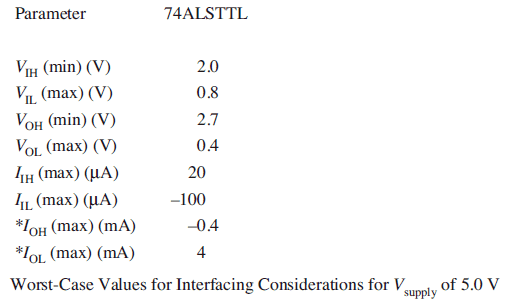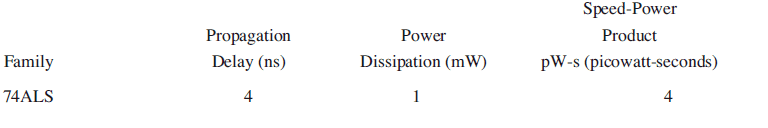## The Attempt at a Solution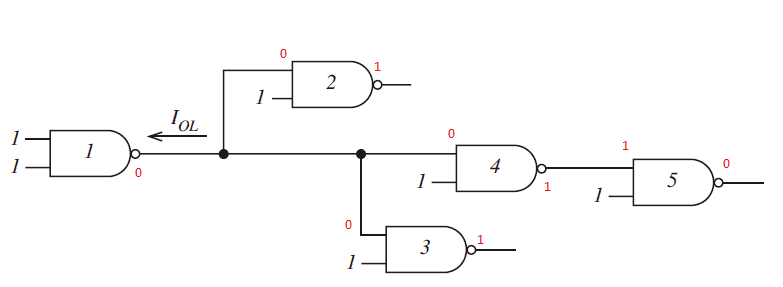I think this a NAND gate logic circuit, in red I have added the inputs and outputs. I have cropped part of a table, that showed typical values of certain logic families, to show the spec of an ALS. I think im right in saying that this can only be an 74ALS. For (a) the low level output according to the table is 4mA, The low level inputs for gates 2,3 and 4 are 100uA each.

I dont know how or where to find the formulas to solve and my notes are dire.

Tom.G
Gold Member
First off, the propagation delay you list doesn't match the data sheet at http://www.ti.com/lit/ds/symlink/sn74als00a.pdf
Be careful reading that data sheet, it covers several devices; not one of their better publications. You might see if there are datasheets available from other mfgs. that are a little easier to follow.

As for formulas, they are almost all 'just' additions. The part that requires thinking is choosing which data sheet line(s) pertain to what you are calculating.

These are the 2 full tables I'm supposed to refer to for this question. How do I estimate IOL? Do i select the best answer from the data sheet?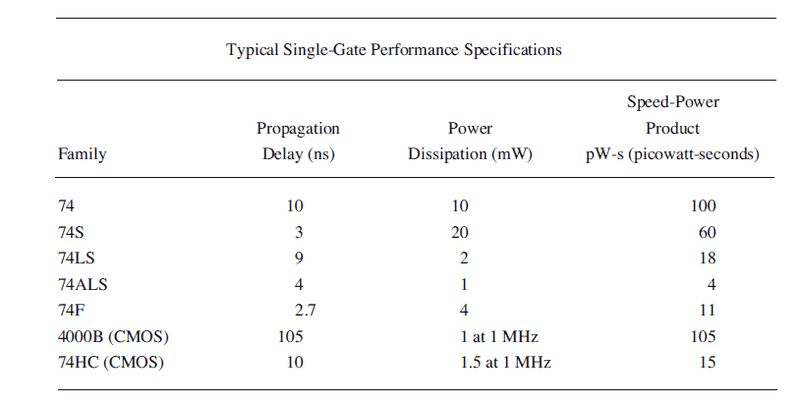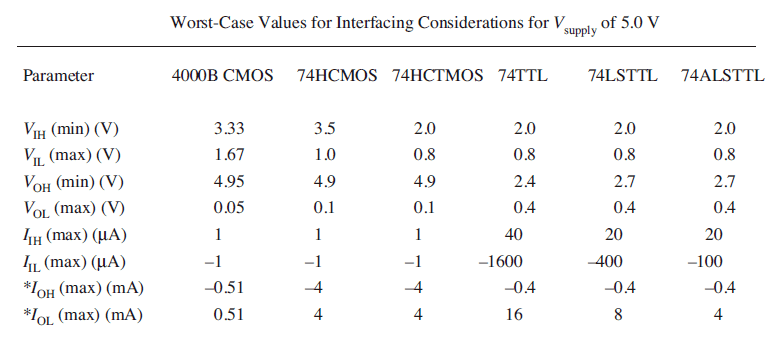Tom.G
Gold Member
If that's what they supplied, that's what you use.a) How many loads, at what current, are supplied by IOL?
b) How many gates does a signal have to travel thru from a GATE 1 input to GATE 5 output?
c) I'll let you think about that one a little more.

Using the ALS figures from the tables

(a) I can see three gate inputs supplied by the output of gate 1. The three gate inputs of gates 2,3 and 4 are LOW and at 100uA each so IOl at gate 1 is 300uA or 0.3mA.

(b) Propagation delay per gate is calculated as (t1+t2)/2 where it switches from HIGH to LOW or vice versa. From the input of gate 1 through gate 4 and to the ouput of gate 5, 3*((4nS+4nS)/2)= 12nS. Although the time take from LOW to HIGH is different from HIGH to LOW

(c) Seems too obvious but for 1mW per gate with 5 gates, total power in a steady state is 5mW.

All seems a bit easy which is why I'm cynical about it all.

Tom.G
Gold Member
(a) Right on.
(b) Right on. Including you observation about the transition times. (Some people miss that unless it's pointed out. Here it's a case of '...that's what they supplied...')
(c) Close. I left the hard one for you here. What about the contribution to power by the gate Input currents? (Although considering the problem statement context, it is unclear if they expect that to be included. Personally, I would. In a large system, that could affect the power supply rating and the cooling requirements. As a real-world rule-of-thumb you can assume that on average half of the inputs will be high and half of them low at a given time.) Watch out, we will probably have another pass at this one.

The trouble I have is calculating power for one gate input using P=IV, when the VIH is a minimum value.

For a HIGH input power, 2*20*10^-6= 0.04. For 7 HIGH inputs that is 0.28mW

For the 3 LOW inputs, (100*10^-6*0.8)*3= 0.24mW ....however

However the Power dissipated or PD=supply voltage Vcc multiplied by the average supply current Icc(avg)

Quotes I have found: " The amount of power dissipated in an IC. It is determined by the current, ICC, that it
draws from the VCC supply, and is given by VCC * ICC . ICC is the average value of ICC (0) and
ICC (1). This power is specified in milliwatts."

"High-level supply current, ICC (1): This is the supply current
when the output of the gate is at logic 1.
Low-level supply current, ICC (0): This is the supply current
when the output of the gate is at logic (0)."

The values are supplied are for 5V (VCC)
If the input is the supply current then ICC(0)=100uA and for 3 LOW inputs that is 300uA

ICC(1)=20uA and for 7 HIGH inputs that is 140uA

Multiplying both by 5V gives us 1.5mW and 0.7mW for a total of 2.2mW

If as you say the rule of thumb in a steady state is 50/50 then: 500uA * 5V=2.5mW and 100uA * 5V= 0.5mW for a total of 3mW

Unless I have the ICC values wrong! :)

Tom.G
Gold Member
You are correct that the power calc be based on supply current, ICC. That is why I said previously '...we will probably have another pass at this one.'

At least one mfg. (http://bitsavers.trailing-edge.com/pdf/signetics/_dataBooks/1989_ALS_Data_Manual.pdf pg4-9 and pg5-4) shows gate power can be anywhere between 600uW and 4mW. Overall, the signal input currents tend to show up in the data sheets as being included in the ICCH and ICCL currents, or sometimes as a worst case value of ICC. Since the data supplied for the problem gave only a single value for power, I would say that's what you have to use (perhaps adding a note that dissipation varies depending on output state.)

Sorry if this seems a little like a run-around, it's not meant to be. I, also, had to refresh my memory from the literature.

Cheers for the input. One last thing, the value given is a quiescent power dissipation value? I don't think its dynamic. If it is quiescent then it has to 5mW total.

Tom.G
•